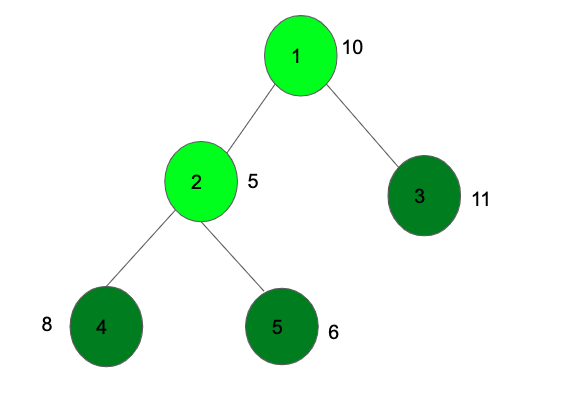# Maximum sum of nodes in Binary tree such that no two are adjacent | Dynamic Programming

Given a N-ary tree with a value associated with each node, the task is to choose a subset of these nodes such that sum of chosen nodes is maximum under a constraint that no two chosen node in subset should be directly connected that is, if we have taken a node in our sum then we can’t take its any children in consideration and vice versa.

Examples:The above diagram selects the nodes with a deep green color to get the maximum value 25.

## Recommended: Please try your approach on {IDE} first, before moving on to the solution.

An approach for this problem has been discussed in the previous post using recursion.

In this post, we will be discussing an approach using Dynamic Programming on Trees.

While solving the problem, there arise two cases:

1. For a particular node, the maximum sum can be calculated by including the node itself along with nodes from its subtree.
2. Or, the maximum sum is calculated by excluding the current node and including only the nodes from its subtree.

Let us assume:

• dp1[node] to be the maximum possible sum by choosing nodes from the subtree of this node and also including the node.
• And, dp2[node] to be the maximum possible sum by choosing nodes from the subtree of the node and not including the node itself.

The first case, if we include the current node, then its value is added and then we can not include any of its immediate children, hence the summation of dp2[] of all the children will be taken into the count to compute dp1[node]. That is,

dp1[node] = tree[node] + sum(dp2[children1], dp2[children2], …)

The second case, if we do not include the current node, then its value is not added, but the children node can be taken or it cannot be taken, hence the summation of the maximum of both for all the children will be taken into count to compute dp2[node]. That is,

dp2[node] = tree[node] + sum(max(dp1[children1], dp2[children1]), max(dp1[children2], dp2[children2])…)

In the end, the final answer will be the maximum of dp1[root] and dp2[root].

Below is the implementation of the above approach:

 `// C++ program to find maximum sum ` `// of a subset of nodes that are not adjacent ` ` `  `#include ` `using` `namespace` `std; ` ` `  `// Function to find the diameter of the tree ` `// using Dynamic Programming ` `void` `dfs(``int` `node, ``int` `parent, ``int` `dp1[], ``int` `dp2[], ` `                            ``list<``int``>* adj, ``int` `tree[]) ` `{ ` ` `  `    ``int` `sum1 = 0, sum2 = 0; ` ` `  `    ``// Traverse for all children of node ` `    ``for` `(``auto` `i = adj[node].begin(); i != adj[node].end(); ++i) { ` `        ``if` `(*i == parent) ` `            ``continue``; ` ` `  `        ``// Call DFS function again ` `        ``dfs(*i, node, dp1, dp2, adj, tree); ` ` `  `        ``// Include the current node ` `        ``// then donot include the children ` `        ``sum1 += dp2[*i]; ` ` `  `        ``// Donot include current node, ` `        ``// then include children or not include them ` `        ``sum2 += max(dp1[*i], dp2[*i]); ` `    ``} ` ` `  `    ``// Recurrence value ` `    ``dp1[node] = tree[node] + sum1; ` `    ``dp2[node] = sum2; ` `} ` ` `  `/* Driver program to test above functions */` `int` `main() ` `{ ` `    ``int` `n = 5; ` ` `  `    ``/* Constructed tree is  ` `        ``1  ` `        ``/ \  ` `        ``2 3  ` `       ``/ \  ` `       ``4 5 */` `    ``list<``int``>* adj = ``new` `list<``int``>[n + 1]; ` ` `  `    ``/* create undirected edges */` `    ``adj.push_back(2); ` `    ``adj.push_back(1); ` `    ``adj.push_back(3); ` `    ``adj.push_back(1); ` `    ``adj.push_back(4); ` `    ``adj.push_back(2); ` `    ``adj.push_back(5); ` `    ``adj.push_back(2); ` ` `  `    ``// Numbers to node ` `    ``int` `tree[n + 1]; ` `    ``tree = 10; ` `    ``tree = 5; ` `    ``tree = 11; ` `    ``tree = 6; ` `    ``tree = 8; ` ` `  `    ``int` `dp1[n + 1], dp2[n + 1]; ` `    ``memset``(dp1, 0, ``sizeof` `dp1); ` `    ``memset``(dp2, 0, ``sizeof` `dp2); ` ` `  `    ``dfs(1, 1, dp1, dp2, adj, tree); ` ` `  `    ``// Find maximum sum by calling function ` `    ``cout << ``"Maximum sum: "` `         ``<< max(dp1, dp2) << endl; ` `    ``return` `0; ` `} `

Output:

```Maximum sum: 25
```

My Personal Notes arrow_drop_upCheck out this Author's contributed articles.

If you like GeeksforGeeks and would like to contribute, you can also write an article using contribute.geeksforgeeks.org or mail your article to contribute@geeksforgeeks.org. See your article appearing on the GeeksforGeeks main page and help other Geeks.

Please Improve this article if you find anything incorrect by clicking on the "Improve Article" button below.

Article Tags :
Practice Tags :

Be the First to upvote.

Please write to us at contribute@geeksforgeeks.org to report any issue with the above content.